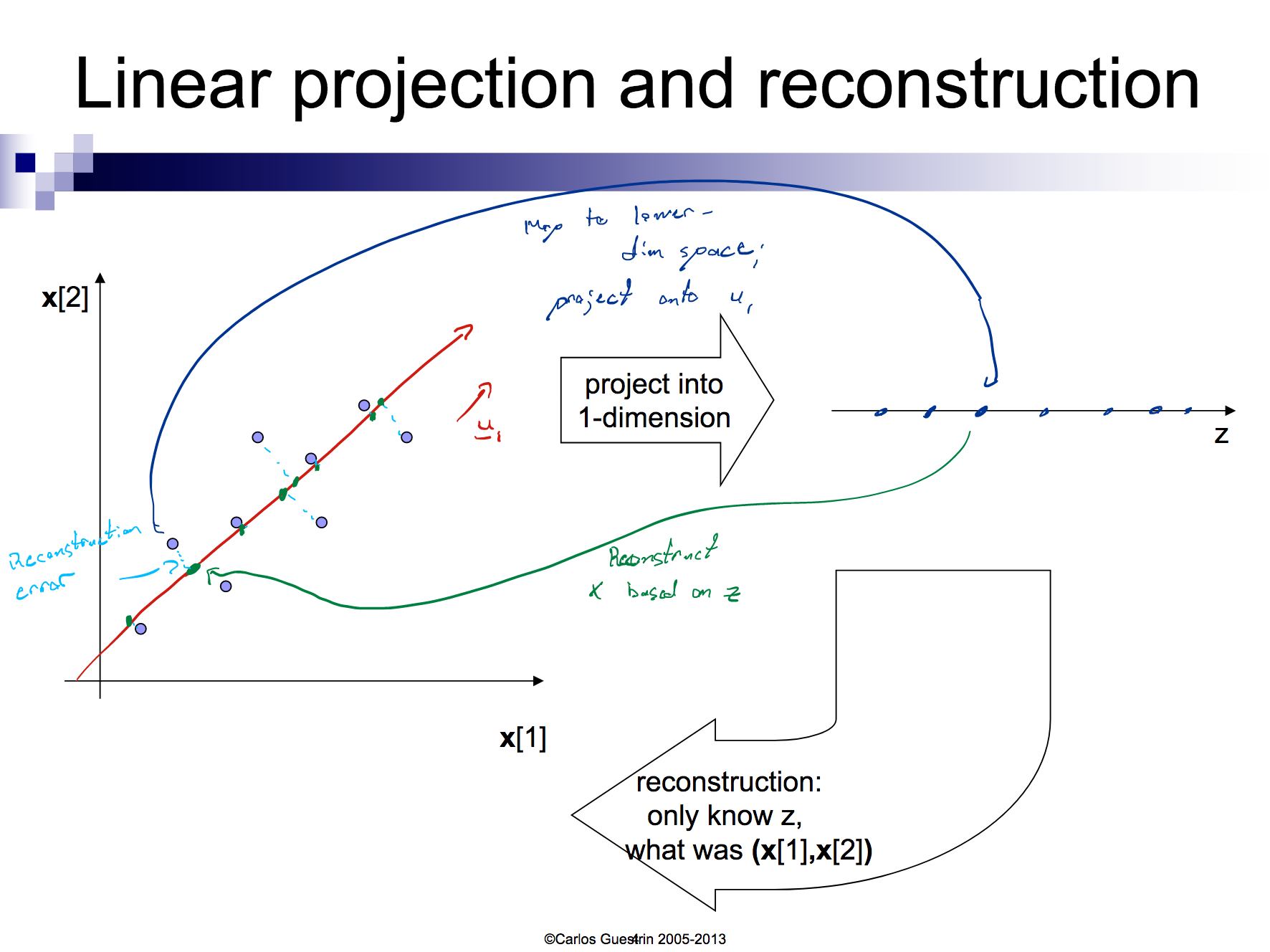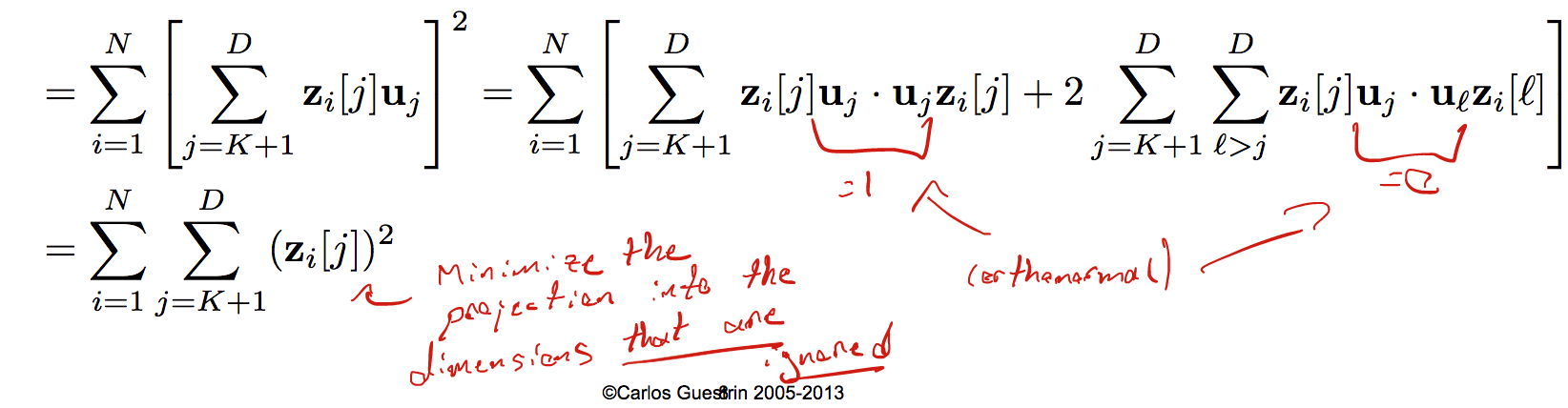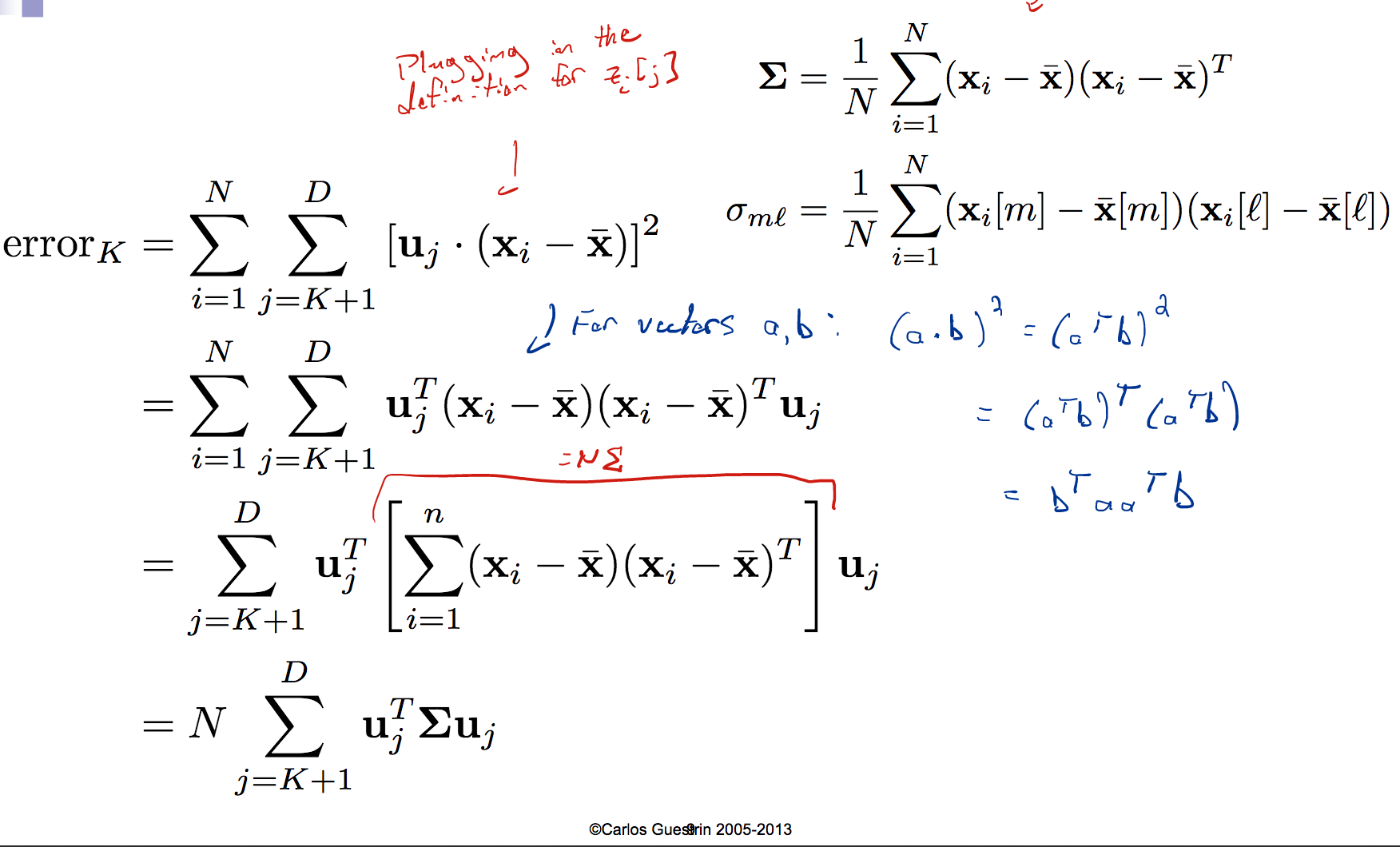# Priniciple Component Analysis (and various Eigen-things)Â¶

#### pspieker@cs.washington.eduÂ¶

Links + places that helped with this notebook:

So we have some dataset with $n$ examples and $d$ dimensions. For some data, $d$ could be large (~10,000 for example), meaning that the data matrix $n\ x\ d$ becomes quite cumbersome to deal with.

Having smaller dimensionality has several more benefits, including:

• making visualization easier (hard to visualize 3D/4D/RD)
• discovering 'intrinsic' dimensionality of the data
• data could actually have many features that are irrelevant

Our goal then could be to reduce the "dimensionality" of the data by significantly reducing the size of $d$. Essentially we want to learn a mapping: $$f: R^{nxd} \rightarrow R^{nxk}$$ with $k << d$.

We want this mapping to also be a "pretty good" representation of the data, so we need to introduce a notion of "goodness" that lets us measure how good a given mapping is.

Loosely, we'll think about our "pretty good" representation as one that is able re-create the original data without much loss of information about it.

### Making this concreteÂ¶

We're also going to do this in the unsupervised setting (we don't have the associated $y$'s).

For simplicity, let's assume I just have a 2 dimensional vector $x = (x_{1}, x_{2})$ that I want to reduce to dimensionality 1.

In :
from IPython.core.display import Image
Image(filename='reconstruction.png', width = 600)

Out:Okay, so we have this idea of projection and then reconstuction:

1. we project that data in the 2 dimensions down into the 1 dimension
2. we reconstruct the data in the 2 dimensions using ONLY the vector we've discovered

Given $n$ data points: $x_1 = (x_{i}, x_{i},...,x_{i}[d])$ for $i=1,...,n$.

We'll represent each point as a projection: $$\hat{x}_{i} = \bar{x} + \sum_{j=1}^{k}\left[z_{i}[j]*u_{j}\right]$$ Note that we can write our original point as $$x_{i} = \bar{x} + \sum_{j=1}^{d}\left[z_{i}[j]*u_{j}\right]$$

Note here that each point has its own $z_{i}[j]$, and that the $u_{[j]}$ are shared for all points. You'll note that we have to keep track of one of these $u_{j}$ for all $j=1,...,k$. Also: $$z_{i}[j] = (x_{i} - \bar{x})\cdot u_{j}$$

### PCAÂ¶

Given k<d, find $(u_{1},...,u_{k})$ that minimize the reconstuction error: $$\text{error}_k = \sum_{i=1}^{n}(x_{i} - \hat{x}_{i})^{2}$$ The $x_i$ here is the truth, and the $\hat{x}_i$ is the reconstructed version.

In linear algebra terms: identify the most meaningful basis to re-express a data set, in the hopes of filtering out noise and reveal hidden structure.

### Understanding the reconstruction errorÂ¶

We'll transform the PCA problem into one you've probably seen before. We'll do this by re-writing the error: $$\text{error}_k = \sum_{i=1}^{n}(x_{i} - \big[\bar{x} + \sum_{j=1}^{k}z_{i}[j]*u_{j}\big])^{2}$$ $$= \sum_{i=1}^{n}\Big[\left[\bar{x} + \sum_{j=1}^{d}z_{i}[j]*u_{j}\right] - \left[\bar{x} + \sum_{j=1}^{k}z_{i}[j]*u_{j}\right]\Big]^{2}$$

In :
from IPython.core.display import Image
Image(filename='reconstruction-math.png', width = 800)

Out:In :
from IPython.core.display import Image
Image(filename='covar.png', width = 800)

Out:So we've completely rewritten our error such that we are: picking an ordered, orthonormal basis $(u_{1},...,u_{d})$ to minimize: $$error_{k} = N \sum_{j=k+1}^{D}u_{j}^{T}\Sigma u_{j}$$

### Eigen-thingsÂ¶

So before we go full Math 308 on you, brief eigen-things review:

Def: eigenvector: A vector $u$ is an eigenvector of some matrix $A$ iff there exists some scalar $\lambda$ such that $Au = \lambda u$.

Def: eigenvalue: the $\lambda$ that corresponds to $u$, so every eigenvector is associated w/an eigenvalue and vice versa.

Def: eigenspace: the set of all eigenvectors corresponding to a given $\lambda$

Quickly doing some algebra: $$Au = \lambda u$$ $$Au - \lambda u = 0$$ $$(A - \lambda I)u = 0$$ So essentially we are looking for non-zero solutions to the above problem. Using the Big Theorem, the above equation has a non-zero solution iff $det(A - \lambda I) = 0$.

Example: Find the eigenvalues for: $$A = \begin{bmatrix} 1 & 0 & 1 \\ 0 & 1 & 2 \\ 0 & 4 & 3 \end{bmatrix}$$ So we solve and recover: $$det(A - \lambda I) = (1- \lambda)(\lambda - 5)(\lambda +1)$$ We have 3 eigenvalues here: $\lambda_{1} = 5$, $\lambda_{2} = 1$, $\lambda_{3} = -1$.

Another way to think about this is minimizing the sum of the $d-k$ eigenvalues of $\Sigma$, or keeping the top $k$ eigenvalues of $\Sigma$.

### The PCA AlgorithmÂ¶

• Start with $n$ by $d$ data matrix $X$
• Recenter: subtract mean from each row of $X$
• Compute the covariance matrix: $\Sigma := \frac{1}{N} X_{c}^{T}X_{c}$
• Find the eigenvalues and eigenvectors of $\Sigma$ (usually via numpy.linalg.eig)
• The principal components: the $k$ eigenvectors with the highest values
In :
from IPython.core.display import Image
Image(filename='eigen-ex.png', width = 1000)

Out: